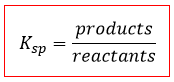# Problem: What concentration of the lead ion, Pb 2+, must be exceeded to precipitate PbF2 from a solution that is 1.00x10-2 M in the fluoride ion, F- =  Ksp for lead(II) fluoride is 3.3x10-8.

###### FREE Expert Solution

For this problem, we´re being asked to calculate the concentration of the lead ion Pb2+ that must be exceeded to precipitate PbF2 from a solution of fluoride ion F-.

In water, the dissociation of PbF2 is the following:

PbF2(s)  Pb2+(aq) + 2 F-(aq)

The Ksp expression for PbF2 is:Where solids are ignored (reactants), so:

${\mathbf{K}}_{\mathbf{sp}}\mathbf{=}\mathbit{p}\mathbit{r}\mathbit{o}\mathbit{d}\mathbit{u}\mathbit{c}\mathbit{t}\mathbit{s}\mathbf{=}\left[{\mathbf{Pb}}^{\mathbf{2}\mathbf{+}}\right]{\left[{\mathbf{F}}^{\mathbf{-}}\right]}^{\mathbf{2}}$

Note that each concentration is raised by the stoichiometric coefficient: [Pb2+] is raised to 1 and [F-] is raised to 2.

92% (198 ratings)###### Problem Details

What concentration of the lead ion, Pb 2+, must be exceeded to precipitate PbF2 from a solution that is 1.00x10-2 M in the fluoride ion, F- =  Ksp for lead(II) fluoride is 3.3x10-8.